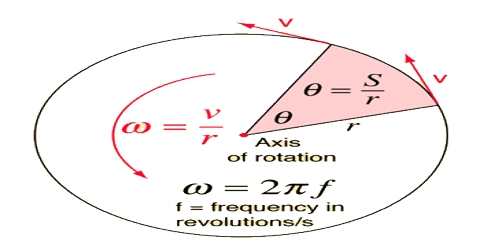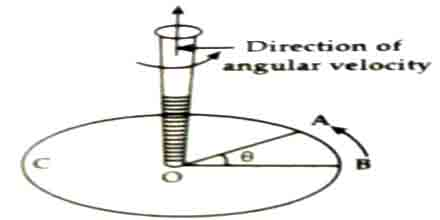# Angular Velocity in Vector Form

Angular velocity

If the time interval approaches zero, the rate of change of angular displacement with time is called instantaneous angular velocity or angular velocity (ώ). Its mathematical expression in vector form is: ῡ = ώ x ř

For an object rotating about an axis, every point on the object has the same angular velocity. Angular velocity has the units rad/s.

Direction of angular velocity (Vector form)

The tangential velocity of any point is proportional to its distance from the axis of rotation. Angular velocity is a vector quantity. It has both direction and magnitude. Its direction can be demonstrated with the help of a right-turn screw.If a particle is rotated in a circular path ABC and by placing the top of a right-turn screw perpendicularly at the center of that plane of the circle is allowed to rotate along the rotation of the particle, then the direction of rotation of the screw will be the angular velocity of the particle (Fig).